Foxtable(狐表)用户栏目专家坐堂 → 按B表循环顺序填充到A表

共有44人关注过本帖树形打印复制链接

# 主题：按B表循环顺序填充到A表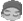1楼 | 信息 | 搜索 | 邮箱 | 主页 | UC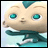加好友发短信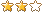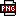此主题相关图片如下：2021-04-08_193737.png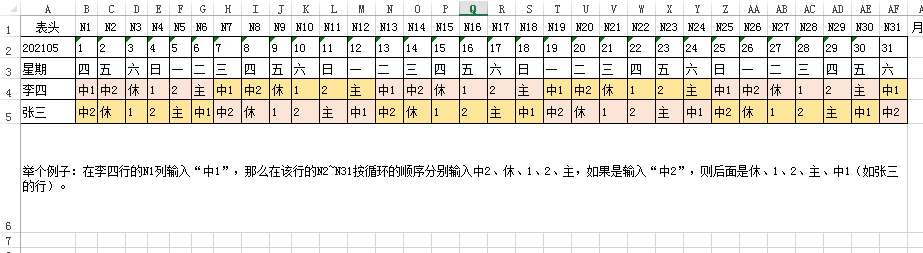B表，即循环顺序表此主题相关图片如下：2021-04-08_193745.png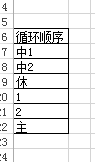自己搞了很久都没有搞好，请教老师，像要实现这样的功能，其代码应该是怎么样写，谢谢。If e.DataCol.Name = "N1" Then    If Forms("增加排班").opened()        MessageBox.Show(0)        Dim nf As String=Forms("增加排班").Controls("ComboBox2").value        Dim yf As String=Forms("增加排班").Controls("ComboBox3").value        Dim ts As Integer=Date.DaysInMonth(nf,yf)        If e.DataRow("N1") = "中1" Then            For i As Integer =2 To ts                    ...........                    ...........                    End If                Next            Next        End If    End IfEnd If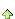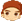2楼 | 信息 | 搜索 | 邮箱 | 主页 | UC加好友发短信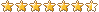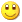Post By：2021/4/8 20:27:00 [只看该作者]

 ……Dim ts As Integer=Date.DaysInMonth(nf,yf)dim s1() as string = {"中1","中2",...}dim idx as integer = array.Indexof(s1, e.DataRow("N1"))if idx = -1 then returnFor i As Integer =2 To tsidx = idx + 1if idx = s1.length then idx = 0      e.DataRow("N" & i) = s1(idx)end ifNext……